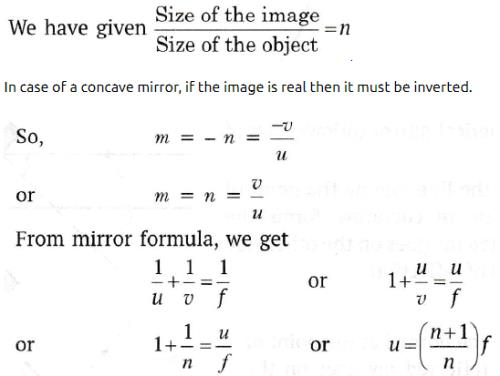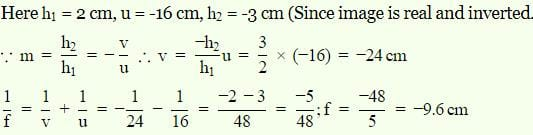UPSC  >  Test: Mirror Formulae

# Test: Mirror Formulae - UPSC

Test Description

## 15 Questions MCQ Test Science & Technology for UPSC CSE - Test: Mirror Formulae

Test: Mirror Formulae for UPSC 2023 is part of Science & Technology for UPSC CSE preparation. The Test: Mirror Formulae questions and answers have been prepared according to the UPSC exam syllabus.The Test: Mirror Formulae MCQs are made for UPSC 2023 Exam. Find important definitions, questions, notes, meanings, examples, exercises, MCQs and online tests for Test: Mirror Formulae below.
Solutions of Test: Mirror Formulae questions in English are available as part of our Science & Technology for UPSC CSE for UPSC & Test: Mirror Formulae solutions in Hindi for Science & Technology for UPSC CSE course. Download more important topics, notes, lectures and mock test series for UPSC Exam by signing up for free. Attempt Test: Mirror Formulae | 15 questions in 15 minutes | Mock test for UPSC preparation | Free important questions MCQ to study Science & Technology for UPSC CSE for UPSC Exam | Download free PDF with solutions
 1 Crore+ students have signed up on EduRev. Have you?
Test: Mirror Formulae - Question 1

### When a mirror is rotated through an angle θ, the reflected ray from it turns through an angle of

Detailed Solution for Test: Mirror Formulae - Question 1

Consider a plane-mirror and a fixed incident ray of light reflecting from the surface at an angle θi. Before the mirror has rotated, the angle of incidence is θ as is the angle of reflection. If the mirror is rotated through an angle φ the normal is rotated by an angle &phi and thus the angle of incidence increases to θ+φ. Therefore, the angle of reflection must also increase by φ to θ+φ The difference between the final angle of reflection and the initial angle of reflection and is 2φ.
Hence, For a fixed incident ray, the angle of the reflection is twice the angle through which the mirror has rotated: thetaf= 2φ

Test: Mirror Formulae - Question 2

### An object is placed 60 cm in front of a concave mirror. The real image formed by the mirror is located 30 cm in front of the mirror. What is the object’s magnification ?

Detailed Solution for Test: Mirror Formulae - Question 2

u = -60 cm, v = -30 cm

m = – v/u = – (-60)/-30 = - 2

Test: Mirror Formulae - Question 3

### A concave mirror of focal length ‘f’ produces an image ‘n’ times farther than the object. If the image is real, then the distance of the object from the mirror is:​

Detailed Solution for Test: Mirror Formulae - Question 3

The correct option is B.Test: Mirror Formulae - Question 4

If the magnification has a negative sign, the image formed by the concave mirror must be

Detailed Solution for Test: Mirror Formulae - Question 4

We know that, if the magnification value is negative sign in the concave mirror, then the image will be real and inverted. Especially, when you come to concave mirror, the images are formed at the left of the mirror. So, it forms real and inverted.

Test: Mirror Formulae - Question 5

If the magnification has a positive sign, the image formed by the concave mirror must be

Detailed Solution for Test: Mirror Formulae - Question 5

It can either be a convex mirror or concave mirror. It depends upon the position of the object. u is always negative so in order to get magnification positive v must be negative. If the object is kept between pole and the focus of the mirror, then it will form a virtual image behind the mirror and v will be +.

Test: Mirror Formulae - Question 6

According to the new cartesian sign convention,

Detailed Solution for Test: Mirror Formulae - Question 6
Whether the focal length of a concave mirror is taken as positive or negative depends on the convention being followed. As per the New Cartesian sign convention, The object is placed on the left of the mirror. Distances are measured from the pole of the mirror.
Test: Mirror Formulae - Question 7

When the image is of double size as the object, the magnification is

Detailed Solution for Test: Mirror Formulae - Question 7

Magnification is the ratio of height of the image to the height of the object. If the image size is double of object size, then the magnification is equal to two.

Test: Mirror Formulae - Question 8

If an object is 14 cm in front of convex mirror and image distance is 5.8 cm then it's focal length is

Detailed Solution for Test: Mirror Formulae - Question 8

The formula for the focal length of a mirror is 1/f = 1/v + 1/u , where f is the focal length, v is the image distance, and u is the object distance.

If we substitute the given values (remembering that for a convex mirror, the object distance is negative), we get:

1/f = 1/(-5.8cm) + 1/(-14cm)

Solving for f, we get f = -4.1 cm.

2. 4.1cm.

Test: Mirror Formulae - Question 9

The mirror formula is valid for

Test: Mirror Formulae - Question 10

When the image is of the same size as the object, the magnification is

Test: Mirror Formulae - Question 11

Which one is correct as the new cartesian sign conventions ?

Detailed Solution for Test: Mirror Formulae - Question 11

Object is always kept on the left side of the mirror therefore its distance is always negative when taken from left of pole.

Test: Mirror Formulae - Question 12

An object is placed on the principal axis of a spherical mirror. The object distance u is:

Test: Mirror Formulae - Question 13

The unit of linear magnification is

Detailed Solution for Test: Mirror Formulae - Question 13

Magnification is thr ratio of size of image to size of object and since it is a ratio of tel similar quantities hence it is unitless.

Test: Mirror Formulae - Question 14

An object 2 cm high is placed at a distance of 16 cm from a concave mirror, which produces a real image 3 cm high. What is the focal length of the mirror ?

Detailed Solution for Test: Mirror Formulae - Question 14

Correct option is A.Test: Mirror Formulae - Question 15

The magnification of a convex mirror is always:

Detailed Solution for Test: Mirror Formulae - Question 15

The image formed by a convex mirror is always smaller in size than the object; hence its magnification is less than 1.

## Science & Technology for UPSC CSE

86 videos|422 docs|225 tests
Information about Test: Mirror Formulae Page
In this test you can find the Exam questions for Test: Mirror Formulae solved & explained in the simplest way possible. Besides giving Questions and answers for Test: Mirror Formulae, EduRev gives you an ample number of Online tests for practice

## Science & Technology for UPSC CSE

86 videos|422 docs|225 tests

### How to Prepare for UPSC

Read our guide to prepare for UPSC which is created by Toppers & the best Teachers(Scan QR code)